Скачать презентацию Circuit Analysis of Circuits Containing Diodes Definition

160b3863344f43a45bbc9ec2fa6791ea.ppt

• Количество слайдов: 20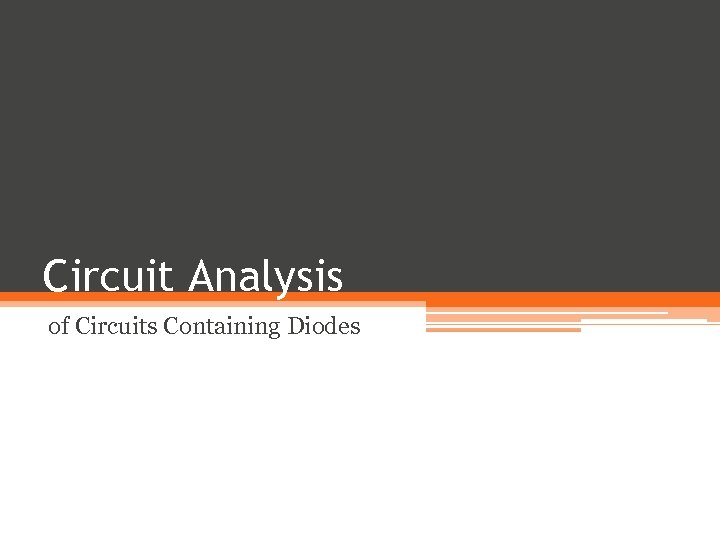Circuit Analysis of Circuits Containing Diodes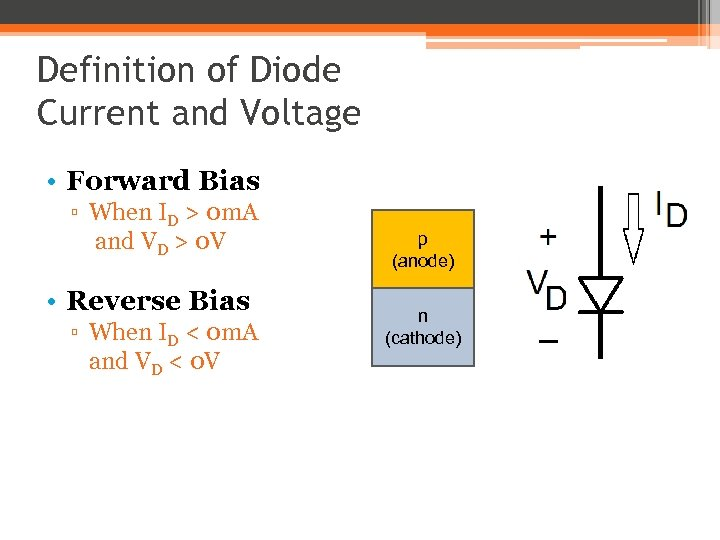Definition of Diode Current and Voltage • Forward Bias ▫ When ID > 0 m. A and VD > 0 V • Reverse Bias ▫ When ID < 0 m. A and VD < 0 V p (anode) n (cathode)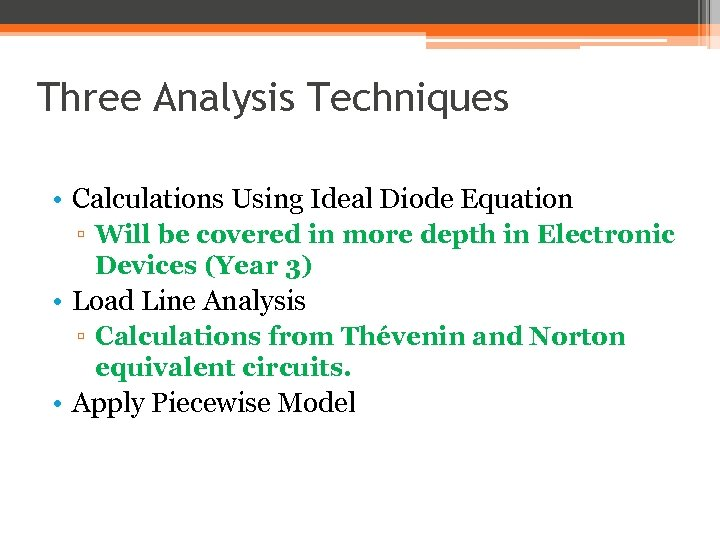Three Analysis Techniques • Calculations Using Ideal Diode Equation ▫ Will be covered in more depth in Electronic Devices (Year 3) • Load Line Analysis ▫ Calculations from Thévenin and Norton equivalent circuits. • Apply Piecewise Model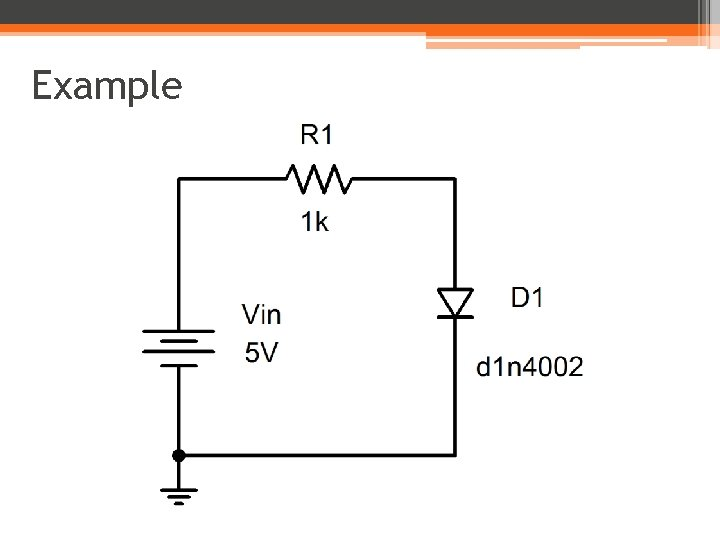Example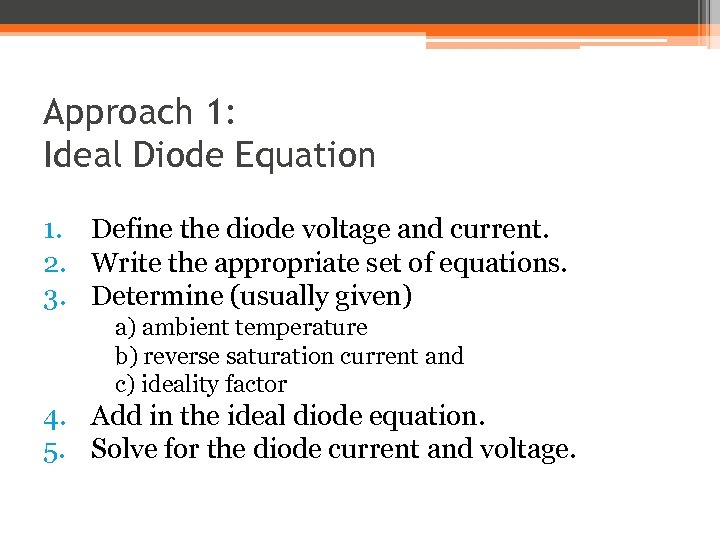Approach 1: Ideal Diode Equation 1. Define the diode voltage and current. 2. Write the appropriate set of equations. 3. Determine (usually given) a) ambient temperature b) reverse saturation current and c) ideality factor 4. Add in the ideal diode equation. 5. Solve for the diode current and voltage.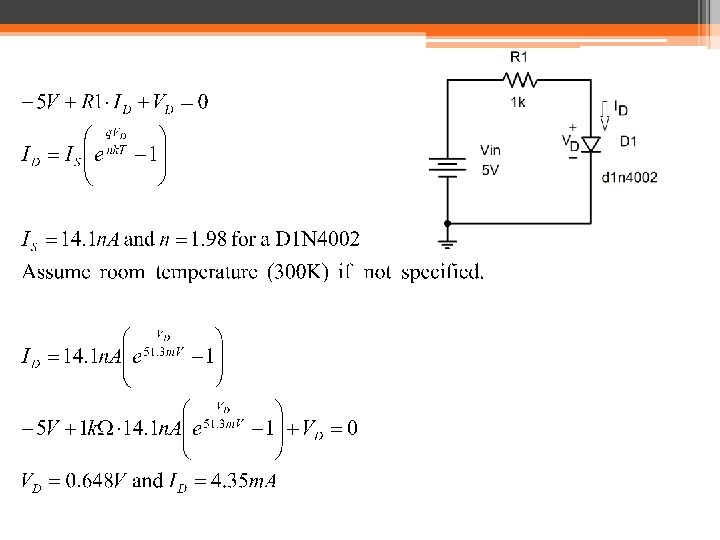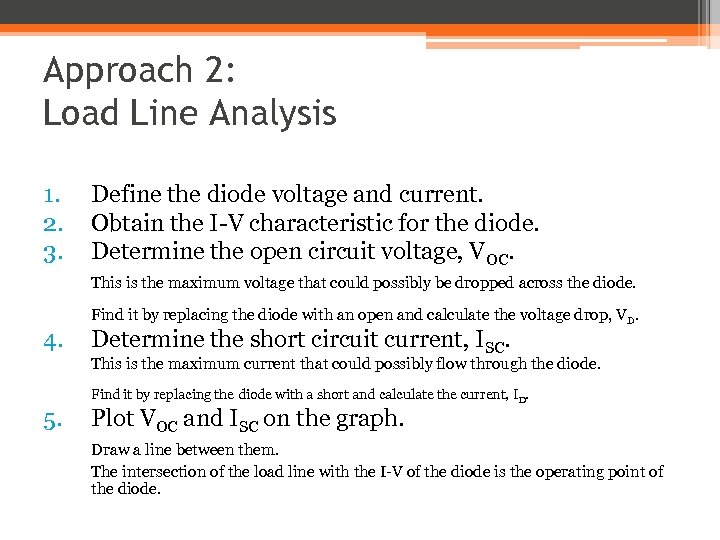Approach 2: Load Line Analysis 1. 2. 3. Define the diode voltage and current. Obtain the I-V characteristic for the diode. Determine the open circuit voltage, VOC. This is the maximum voltage that could possibly be dropped across the diode. 4. Find it by replacing the diode with an open and calculate the voltage drop, VD. Determine the short circuit current, ISC. This is the maximum current that could possibly flow through the diode. 5. Find it by replacing the diode with a short and calculate the current, ID. Plot VOC and ISC on the graph. Draw a line between them. The intersection of the load line with the I-V of the diode is the operating point of the diode.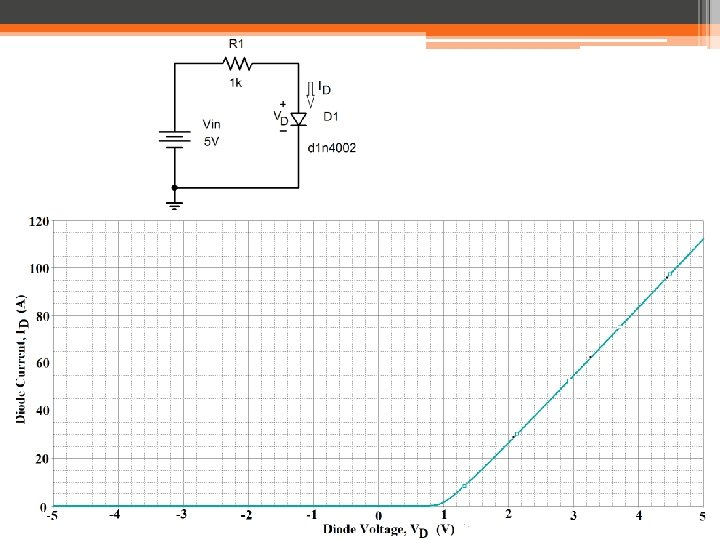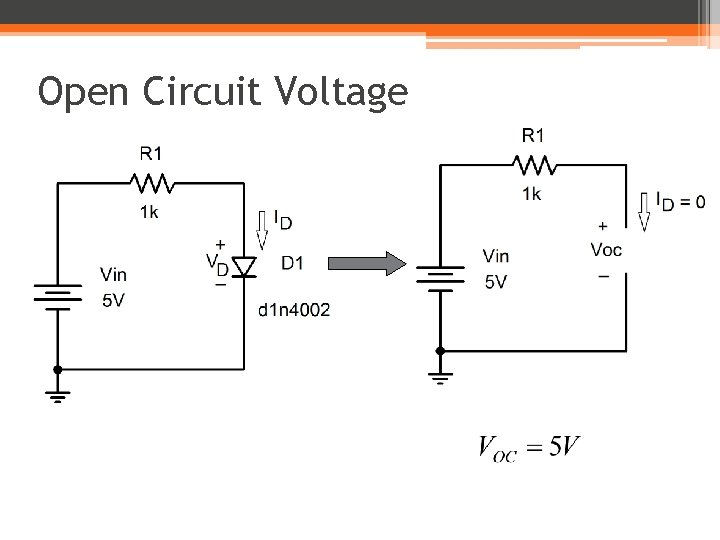Open Circuit Voltage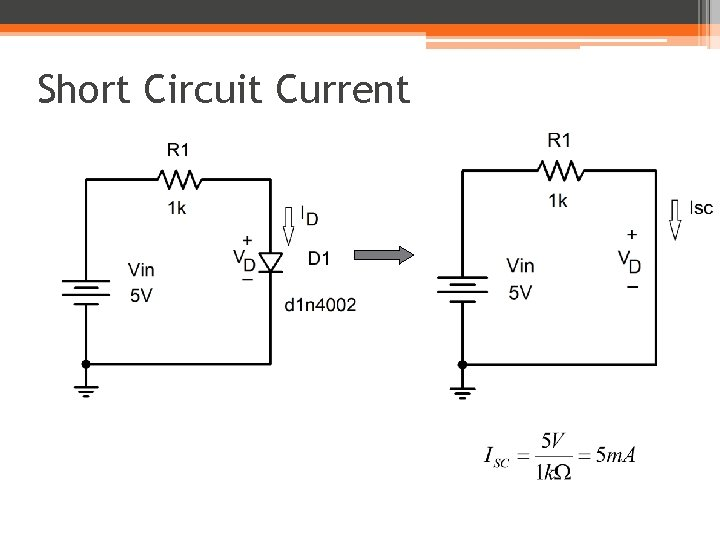Short Circuit Current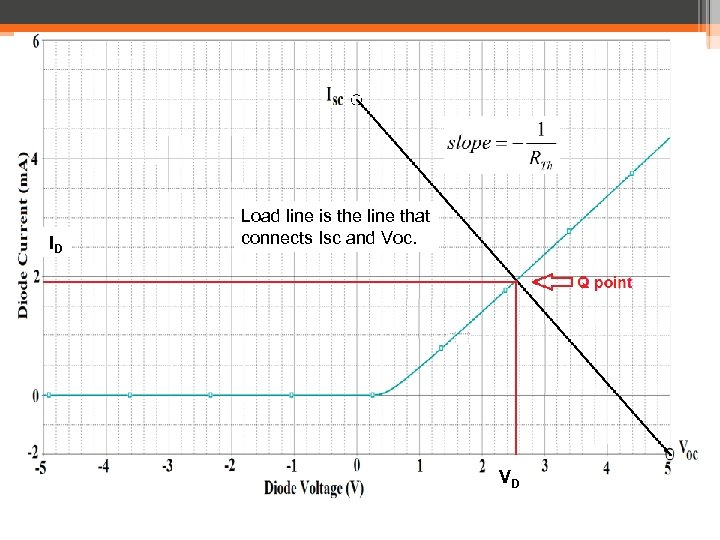ID Load line is the line that connects Isc and Voc. VD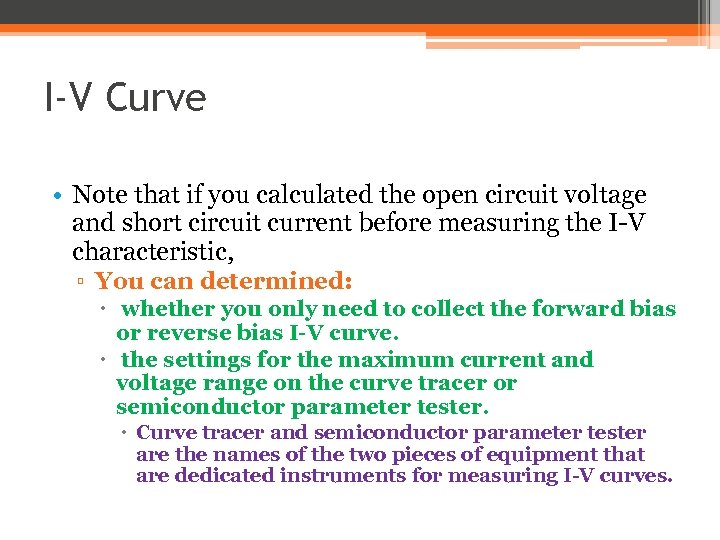I-V Curve • Note that if you calculated the open circuit voltage and short circuit current before measuring the I-V characteristic, ▫ You can determined: whether you only need to collect the forward bias or reverse bias I-V curve. the settings for the maximum current and voltage range on the curve tracer or semiconductor parameter tester. Curve tracer and semiconductor parameter tester are the names of the two pieces of equipment that are dedicated instruments for measuring I-V curves.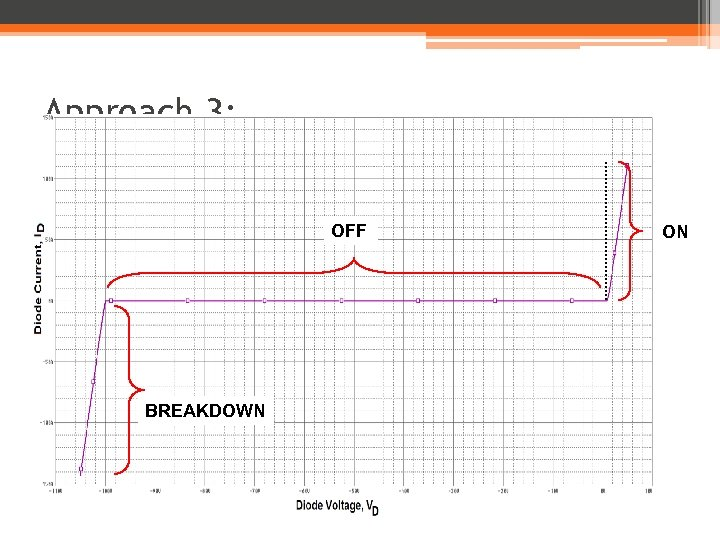Approach 3: Piecewise Models OFF BREAKDOWN ON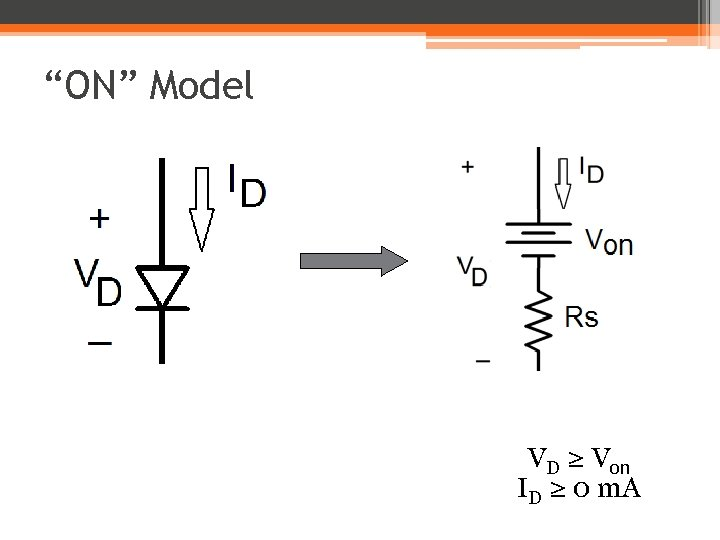“ON” Model VD ≥ Von ID ≥ 0 m. A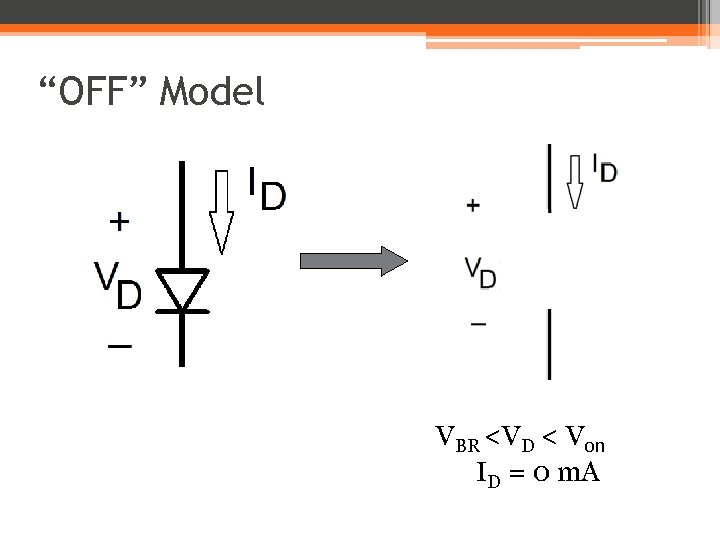“OFF” Model VBR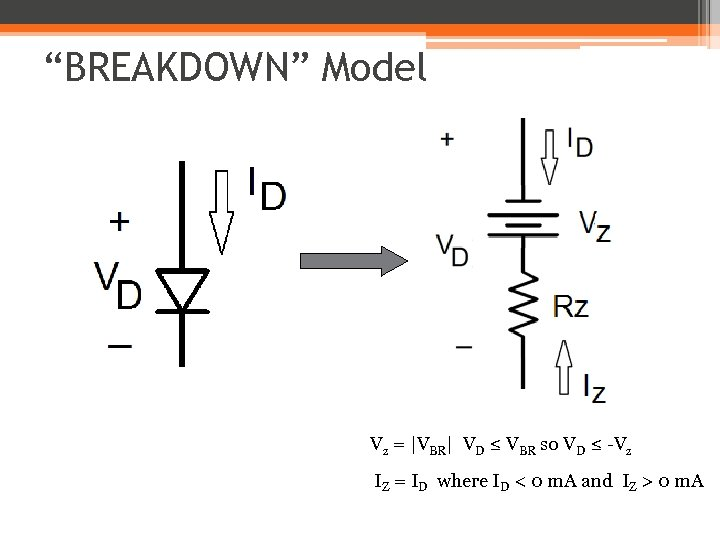“BREAKDOWN” Model Vz = |VBR| VD ≤ VBR so VD ≤ -Vz IZ = ID where ID < 0 m. A and IZ > 0 m. A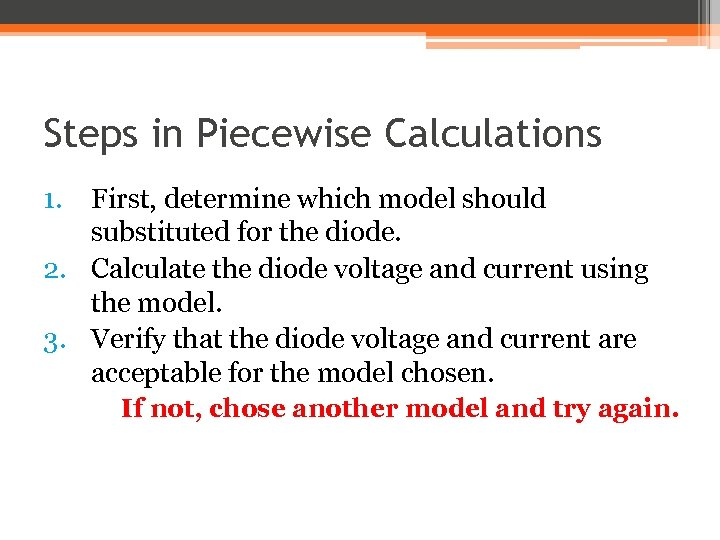Steps in Piecewise Calculations 1. First, determine which model should substituted for the diode. 2. Calculate the diode voltage and current using the model. 3. Verify that the diode voltage and current are acceptable for the model chosen. If not, chose another model and try again.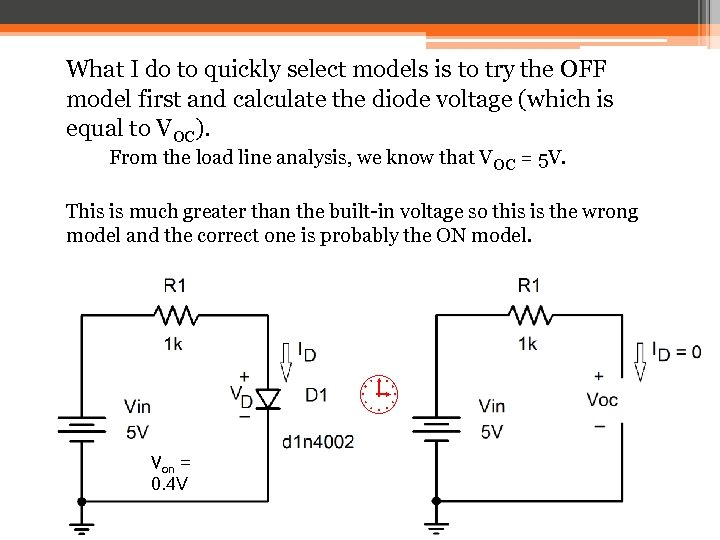What I do to quickly select models is to try the OFF model first and calculate the diode voltage (which is equal to VOC). From the load line analysis, we know that VOC = 5 V. This is much greater than the built-in voltage so this is the wrong model and the correct one is probably the ON model. Von = 0. 4 V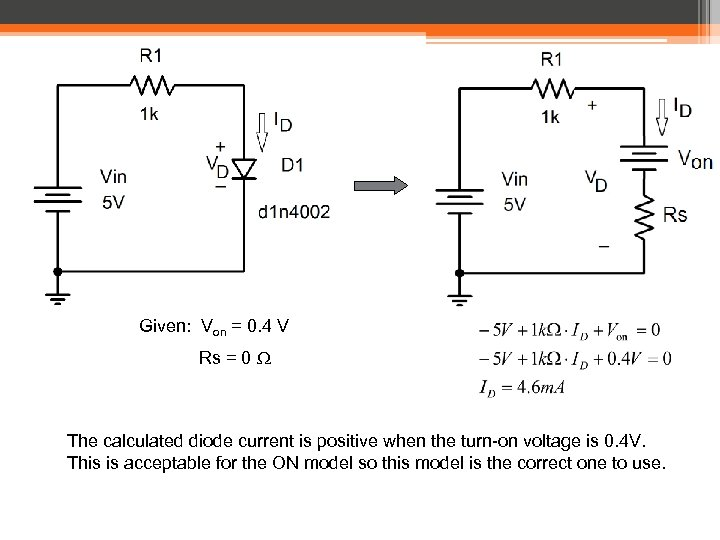Given: Von = 0. 4 V Rs = 0 W The calculated diode current is positive when the turn-on voltage is 0. 4 V. This is acceptable for the ON model so this model is the correct one to use.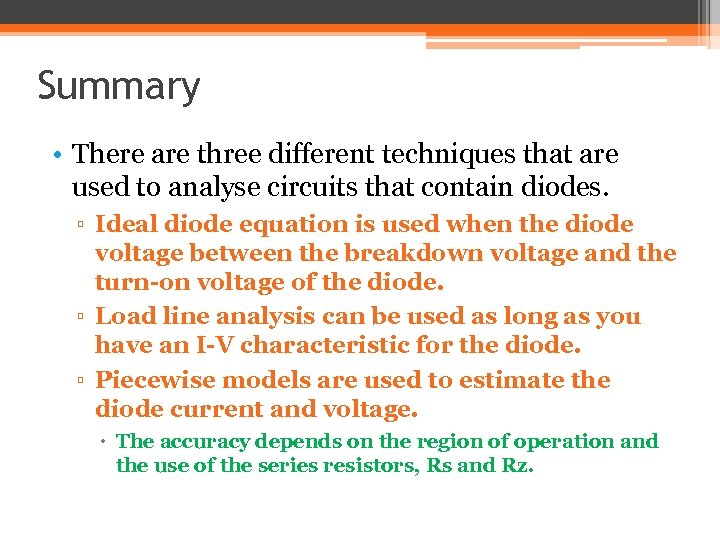Summary • There are three different techniques that are used to analyse circuits that contain diodes. ▫ Ideal diode equation is used when the diode voltage between the breakdown voltage and the turn-on voltage of the diode. ▫ Load line analysis can be used as long as you have an I-V characteristic for the diode. ▫ Piecewise models are used to estimate the diode current and voltage. The accuracy depends on the region of operation and the use of the series resistors, Rs and Rz.